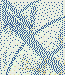Author Message anabela_cGroup: Forum Members Posts: 23, Visits: 75 Hi Dave,I'm trying to customize the distance between two values using a horizontal radiobuttons, so that each text option appears aligned below two images. The only way I found to do the trick was to use a space after the text, as follows:/ options = ("First option            ", "Second option")/ orientation = horizontal/ optionvalues = ("1", "2")/ responsefontstyle = ("Arial", 3%, true)/ size = (50, 10)/ position = (30%, 70%)/ questions = [1=Example]/ stimulusframes = [1 = PartnersPic]/ showbackbutton = false/ showquestionnumbers = false/ showpagenumbers = false/ showmousecursor = true/ navigationbuttonsize = (15,5)/ finishlabel = "Continue"/ nextbuttonposition = (42,90)/ items = ("160200_new.png")/ position = (50%,45%)/ size = (65%,65%)/ trials = [1 = Examplee]/ blocks = [1 = Exa]However, when I tried the experiment on a colleague's computer, the text with the space was a bit hidden below the image (so that "First option" appeared higher than "Second option"). This does not seem to be due to the screen resolution, but at least seems to be fixed when I remove the space after "First option". Therefore, do you know how to adjust the distance between the text, without using the space? Thanks in advance!! anabela_cGroup: Forum Members Posts: 23, Visits: 75 +x anabela_c - 6/9/2022Hi Dave,I'm trying to customize the distance between two values using a horizontal radiobuttons, so that each text option appears aligned below two images. The only way I found to do the trick was to use a space after the text, as follows:/ options = ("First option            ", "Second option")/ orientation = horizontal/ optionvalues = ("1", "2")/ responsefontstyle = ("Arial", 3%, true)/ size = (50, 10)/ position = (30%, 70%)/ questions = [1=Example]/ stimulusframes = [1 = PartnersPic]/ showbackbutton = false/ showquestionnumbers = false/ showpagenumbers = false/ showmousecursor = true/ navigationbuttonsize = (15,5)/ finishlabel = "Continue"/ nextbuttonposition = (42,90)/ items = ("160200_new.png")/ position = (50%,45%)/ size = (65%,65%)/ trials = [1 = Examplee]/ blocks = [1 = Exa]However, when I tried the experiment on a colleague's computer, the text with the space was a bit hidden below the image (so that "First option" appeared higher than "Second option"). This does not seem to be due to the screen resolution, but at least seems to be fixed when I remove the space after "First option". Therefore, do you know how to adjust the distance between the text, without using the space? Thanks in advance!!Daveposted Last Year ANSWERGroup: Administrators Posts: 12K, Visits: 91K +x anabela_c - 6/9/2022+x anabela_c - 6/9/2022Hi Dave,I'm trying to customize the distance between two values using a horizontal radiobuttons, so that each text option appears aligned below two images. The only way I found to do the trick was to use a space after the text, as follows:/ options = ("First option            ", "Second option")/ orientation = horizontal/ optionvalues = ("1", "2")/ responsefontstyle = ("Arial", 3%, true)/ size = (50, 10)/ position = (30%, 70%)/ questions = [1=Example]/ stimulusframes = [1 = PartnersPic]/ showbackbutton = false/ showquestionnumbers = false/ showpagenumbers = false/ showmousecursor = true/ navigationbuttonsize = (15,5)/ finishlabel = "Continue"/ nextbuttonposition = (42,90)/ items = ("160200_new.png")/ position = (50%,45%)/ size = (65%,65%)/ trials = [1 = Examplee]/ blocks = [1 = Exa]However, when I tried the experiment on a colleague's computer, the text with the space was a bit hidden below the image (so that "First option" appeared higher than "Second option"). This does not seem to be due to the screen resolution, but at least seems to be fixed when I remove the space after "First option". Therefore, do you know how to adjust the distance between the text, without using the space? Thanks in advance!!There's no way to manipulate the distance between options other than padding the length using spaces or similar (e.g. tabs, that is ~t; https://www.millisecond.com/support/docs/v5/html/language/specialchars.htm ). In addition, you'll want to specify a consistent /canvasaspectratio in to avoid alignment issues due to varying resolutions and aspect ratios. https://www.millisecond.com/support/docs/v5/html/language/attributes/canvasaspectratio.htm anabela_cGroup: Forum Members Posts: 23, Visits: 75 +x Dave - 6/9/2022+x anabela_c - 6/9/2022+x anabela_c - 6/9/2022Hi Dave,I'm trying to customize the distance between two values using a horizontal radiobuttons, so that each text option appears aligned below two images. The only way I found to do the trick was to use a space after the text, as follows:/ options = ("First option            ", "Second option")/ orientation = horizontal/ optionvalues = ("1", "2")/ responsefontstyle = ("Arial", 3%, true)/ size = (50, 10)/ position = (30%, 70%)/ questions = [1=Example]/ stimulusframes = [1 = PartnersPic]/ showbackbutton = false/ showquestionnumbers = false/ showpagenumbers = false/ showmousecursor = true/ navigationbuttonsize = (15,5)/ finishlabel = "Continue"/ nextbuttonposition = (42,90)/ items = ("160200_new.png")/ position = (50%,45%)/ size = (65%,65%)/ trials = [1 = Examplee]/ blocks = [1 = Exa]However, when I tried the experiment on a colleague's computer, the text with the space was a bit hidden below the image (so that "First option" appeared higher than "Second option"). This does not seem to be due to the screen resolution, but at least seems to be fixed when I remove the space after "First option". Therefore, do you know how to adjust the distance between the text, without using the space? Thanks in advance!!There's no way to manipulate the distance between options other than padding the length using spaces or similar (e.g. tabs, that is ~t; https://www.millisecond.com/support/docs/v5/html/language/specialchars.htm ). In addition, you'll want to specify a consistent /canvasaspectratio in to avoid alignment issues due to varying resolutions and aspect ratios. https://www.millisecond.com/support/docs/v5/html/language/attributes/canvasaspectratio.htmthanks a lot!

#### Merge Selected

Merge into selected topic...

Merge into merge target...

Merge into a specific topic ID...